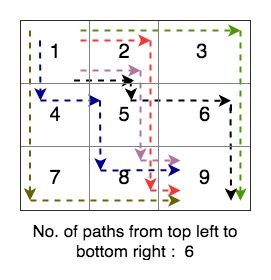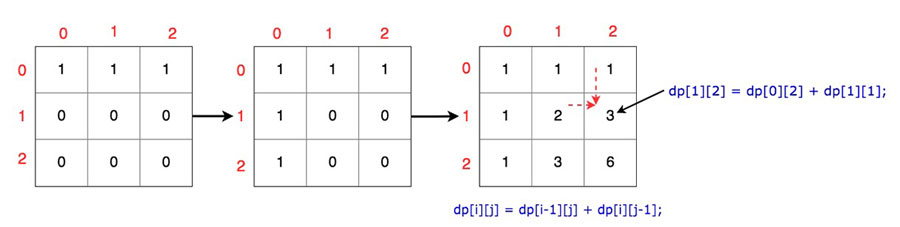# Count all paths from top left to bottom right of MxN matrix

In this post, we will see about how to count all paths from top left to bottom right of MxN matrix.

## Problem

We need to count all paths from top left to bottom right of MxN matrix. You can either move down or right.## Solution

You can solve this problem using recursion.

### Recursion

Recursion will work fine but time complexity of this solution will be exponential as there are lots of overlapping subproblems here.

We can use dynamic programming to solve the problem. We won’t recompute any subproblem more than once.

### Dynamic programming

Here is simple algorithm for dynamic programming solution.

• Initialize an array dp with matrix’s dimensions. This array will give us final count, once we reach to bottom right.
• Fill the first row with 1, as you can reach here from one direction(by going right)
• Fill the first column with 1 as well, as you can reach here from one direction(by going down).
• Iterate over all other rows and columns and use formula dp=dp[i-1][j] + dp[i][j-1], as you can reach here from two directions (By going right or By going down)
• return dp[matrix.length-1][matrix.length-1]

Here is a diagramtic illustration of the algorithm.Here is complete program to Count all paths from top left to bottom right of MxN matrix.

Here is output of the above program.

Total paths to reach top left to bottom right using recursion: 6
Total paths to reach top left to bottom right using DP: 6

That’s all about counting all paths from top left to bottom right of MxN matrix.